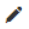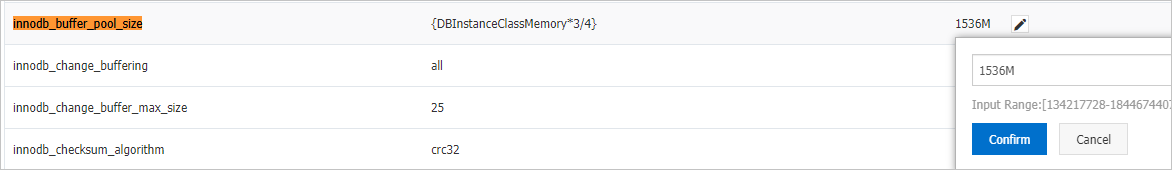This topic describes how to configure the innodb_buffer_pool_size parameter of an ApsaraDB RDS for MariaDB TX instance based on your business requirements. This way, you can improve the performance of the instance.

## Background information

You can reconfigure the innodb_buffer_pool_size parameter to adjust the size of the InnoDB buffer pool for an RDS instance. The value of this parameter must be calculated by using the following formula:

``{DBInstanceClassMemory*X/Y}    ``

Example:

``{DBInstanceClassMemory*7/10}``
Note
• DBInstanceClassMemory is a system variable, which specifies the memory capacity of the RDS instance.
• X is the numerator, and Y is the denominator.
• The size of the InnoDB buffer pool must be within the following range: [128 MB, DBInstanceClassMemory × 8/10]. The minimum size is 128 MB, and the maximum size is 80% of the memory capacity that you purchased for the RDS instance.

The default size of the InnoDB buffer pool for an RDS instance is calculated based on the following rules:

• If the RDS instance is equipped with standard SSDs or enhanced SSDs (ESSDs) and the `purchased memory capacity of the RDS instance is less than 16 GB`, the default size of the InnoDB buffer pool is calculated by using the following formula: ```Default size of the InnoDB buffer pool = (Purchased memory capacity of the RDS instance - Reserved memory capacity of the RDS instance) × 0.75```.
Note The reserved memory capacity of the RDS instance is calculated by using the following formula:
``MIN{Purchased memory capacity of the RDS instance × 0.65, [(Purchased memory capacity of the RDS instance/16384) + 1] × 2048}``
• If the RDS instance is equipped with standard SSDs or ESSDs and the `purchased memory capacity of the RDS instance is greater than or equal to 16 GB`, the default size of the InnoDB buffer pool is calculated by using the following formula: ```Default size of the InnoDB buffer pool = Purchased memory capacity of the RDS instance × 0.75```.
Note The default size of the InnoDB buffer pool is an integer multiple of 128. If the calculated result is not an integer multiple of 128, an approximate integer that is an integer multiple of 128 is taken. For example, an RDS instance provides 1,024 MB of memory, the calculated result is 268, and the approximate integer that is a multiple of 128 is 256. In this case, the default size of the InnoDB buffer pool for the RDS instance is 256 MB.

The following table provides the default size and the maximum size of the InnoDB buffer pool for various memory capacities.

Memory capacity (Unit: MB) Default buffer pool size (Unit: MB) Maximum buffer pool size (Unit: MB)
2,048 512 512
4,096 1,536 1,536
8,192 4,608 4,608
16,384 12,288 12,288
32,768 24,576 25,600
65,536 49,152 52,224
131,072 98,304 104,448
196,608 147,456 156,672
229,376 172,032 183,296
262,144 196,608 208,896
491,520 368,640 393,216

The size of the InnoDB buffer pool must be a multiple of the result that is obtained by using the following formula: ```Value of the innodb_buffer_pool_chunk_size parameter × Value of the innodb_buffer_pool_instances parameter```. If the size of the InnoDB buffer pool is not a multiple of the result that you obtain by using the formula, ApsaraDB RDS changes the size to a multiple of the result. For example, if the result that you obtain by using the formula is 1 GB and you set the `innodb_buffer_pool_size` parameter to 1.5 GB, ApsaraDB changes the value of the innodb_buffer_pool_size parameter to 2 GB.

## Procedure

1. Visit the RDS instance list, select a region above, and click the target instance ID.
2. In the left-side navigation pane, click Parameters.
3. Find the innodb_buffer_pool_size parameter and click theicon. In the dialog box that appears, enter a new value and click OK.
Warning After you change the value of the innodb_buffer_pool_size parameter for an RDS instance, the instance restarts. Proceed with caution.4. Click Apply Changes above the parameter list. In the message that appears, click OK. Then, wait for the RDS instance to restart.